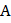# On a two-lane road, caris travelling with a speed of. Two carsandapproach carin opposite directions with a speed ofeach. At a certain instant, when the distanceis equal to, both being 1 km,decides to overtakesbeforedoes. What minimum acceleration of caris required to avoid an accident?

## Question ID - 153859 :- On a two-lane road, caris travelling with a speed of. Two carsandapproach carin opposite directions with a speed ofeach. At a certain instant, when the distanceis equal to, both being 1 km,decides to overtakesbeforedoes. What minimum acceleration of caris required to avoid an accident?

3537

(1)

The situation can be roughly shown in the figure. Lettake timeto overtakeHereLet acceleration ofbefor overtakingands

UsingNext Question :
 A police jeep is chasing a culprit going on a motorbike. The motorbike crosses a turning at a speed of. The jeep follows it at a speed of, crossing the turning 10 s later than the bike Assuming that they travel at constant speeds, how far from the turning will the jeep catch up with the bike? (In km)